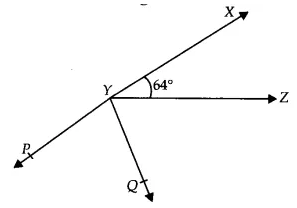Q

# It is given that anlge XYZ = 64 degrees and XY is produced to point P. Draw a figure from the given information. If ray YQ bisects anlge ZYP, find anlge XYQ and reflex anlge QYP.

6. It is given that XYZ = 64° and XY is produced to point P. Draw a figure from the given information. If ray YQ bisects ZYP, find XYQ and reflex QYP.

ViewsGiven that,
XYZ =  and XY produced to point P and Ray YQ bisects ZYP
Now, XYP is a straight line
So, XYZ + ZYQ + QYP =

Thus reflex of QYP =

Since XYQ = XYZ + ZYQ  [

Exams
Articles
Questions